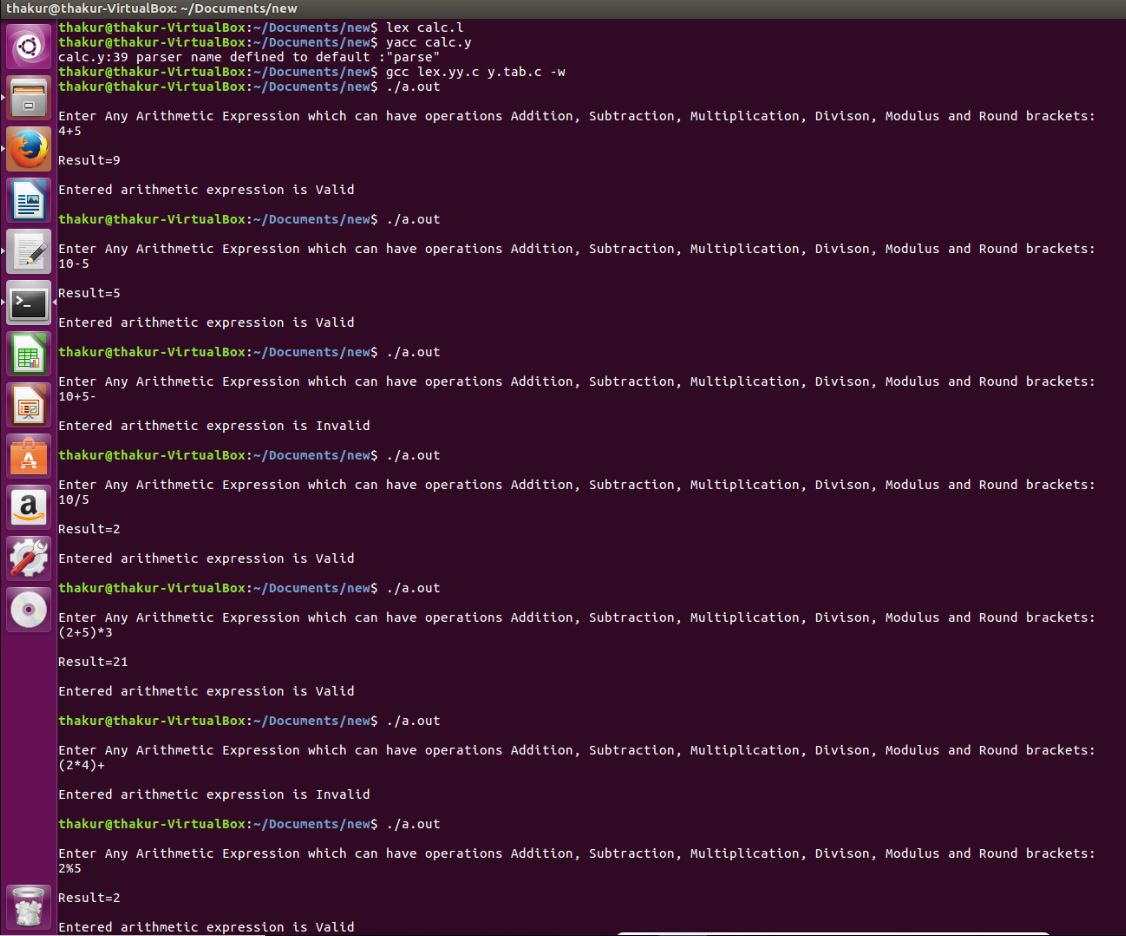# YACC program to implement a Calculator and recognize a valid Arithmetic expression

Problem: YACC program to implement a Calculator and recognize a valid Arithmetic expression.

Explanation:
Yacc (for “yet another compiler compiler.”) is the standard parser generator for the Unix operating system. An open source program, yacc generates code for the parser in the C programming language. The acronym is usually rendered in lowercase but is occasionally seen as YACC or Yacc.

Examples:

```Input: 4+5
Output: Result=9
Entered arithmetic expression is Valid

Input: 10-5
Output: Result=5
Entered arithmetic expression is Valid

Input: 10+5-
Output:
Entered arithmetic expression is Invalid

Input: 10/5
Output: Result=2
Entered arithmetic expression is Valid

Input: (2+5)*3
Output: Result=21
Entered arithmetic expression is Valid

Input: (2*4)+
Output:
Entered arithmetic expression is Invalid

Input: 2%5
Output: Result=2
Entered arithmetic expression is Valid ```

Lexical Analyzer Source Code:

 `%{ ` `   ``/* Definition section */` `  ``#include ` `  ``#include "y.tab.h" ` `  ``extern` `int` `yylval; ` `%} ` ` `  `/* Rule Section */` `%% ` `[0-9]+ { ` `          ``yylval=``atoi``(yytext); ` `          ``return` `NUMBER; ` ` `  `       ``} ` `[\t] ; ` ` `  `[\n] ``return` `0; ` ` `  `. ``return` `yytext; ` ` `  `%% ` ` `  `int` `yywrap() ` `{ ` ` ``return` `1; ` `} `

Parser Source Code :

 `%{ ` `   ``/* Definition section */` `  ``#include ` `  ``int` `flag=0; ` `%} ` ` `  `%token NUMBER ` ` `  `%left ``'+'` `'-'` ` `  `%left ``'*'` `'/'` `'%'` ` `  `%left ``'('` `')'` ` `  `/* Rule Section */` `%% ` ` `  `ArithmeticExpression: E{ ` ` `  `         ``printf``(``"\nResult=%d\n"``, \$\$); ` ` `  `         ``return` `0; ` ` `  `        ``}; ` ` ``E:E``'+'``E {\$\$=\$1+\$3;} ` ` `  ` ``|E``'-'``E {\$\$=\$1-\$3;} ` ` `  ` ``|E``'*'``E {\$\$=\$1*\$3;} ` ` `  ` ``|E``'/'``E {\$\$=\$1/\$3;} ` ` `  ` ``|E``'%'``E {\$\$=\$1%\$3;} ` ` `  ` ``|``'('``E``')'` `{\$\$=\$2;} ` ` `  ` ``| NUMBER {\$\$=\$1;} ` ` `  ` ``; ` ` `  `%% ` ` `  `//driver code ` `void` `main() ` `{ ` `   ``printf``("\nEnter Any Arithmetic Expression which  ` `                   ``can have operations Addition,  ` `                   ``Subtraction, Multiplication, Divison,  ` `                          ``Modulus and Round brackets:\n"); ` ` `  `   ``yyparse(); ` `   ``if``(flag==0) ` `   ``printf``(``"\nEntered arithmetic expression is Valid\n\n"``); ` `} ` ` `  `void` `yyerror() ` `{ ` `   ``printf``(``"\nEntered arithmetic expression is Invalid\n\n"``); ` `   ``flag=1; ` `} `

Output:GeeksforGeeks has prepared a complete interview preparation course with premium videos, theory, practice problems, TA support and many more features. Please refer Placement 100 for details

My Personal Notes arrow_drop_upCheck out this Author's contributed articles.

If you like GeeksforGeeks and would like to contribute, you can also write an article using contribute.geeksforgeeks.org or mail your article to contribute@geeksforgeeks.org. See your article appearing on the GeeksforGeeks main page and help other Geeks.

Please Improve this article if you find anything incorrect by clicking on the "Improve Article" button below.

Article Tags :

Be the First to upvote.

Please write to us at contribute@geeksforgeeks.org to report any issue with the above content.